NEET  >  Test: Chemistry - 12

# Test: Chemistry - 12

Test Description

## 45 Questions MCQ Test NEET Mock Test Series | Test: Chemistry - 12

Test: Chemistry - 12 for NEET 2023 is part of NEET Mock Test Series preparation. The Test: Chemistry - 12 questions and answers have been prepared according to the NEET exam syllabus.The Test: Chemistry - 12 MCQs are made for NEET 2023 Exam. Find important definitions, questions, notes, meanings, examples, exercises, MCQs and online tests for Test: Chemistry - 12 below.
Solutions of Test: Chemistry - 12 questions in English are available as part of our NEET Mock Test Series for NEET & Test: Chemistry - 12 solutions in Hindi for NEET Mock Test Series course. Download more important topics, notes, lectures and mock test series for NEET Exam by signing up for free. Attempt Test: Chemistry - 12 | 45 questions in 45 minutes | Mock test for NEET preparation | Free important questions MCQ to study NEET Mock Test Series for NEET Exam | Download free PDF with solutions
 1 Crore+ students have signed up on EduRev. Have you?
Test: Chemistry - 12 - Question 1

### An alkylmagnesium halide reacts with water to give an alkane. In this reaction water acts as

Test: Chemistry - 12 - Question 2

### The reactivity of the halogens towards methane decreases in the order

Test: Chemistry - 12 - Question 3

### Which of the following statements is correct in relation to the halogenation of alkanes ?

Test: Chemistry - 12 - Question 4
Which of the following reactions will lead to the creation of two chiral centers in the product ?
Test: Chemistry - 12 - Question 5
trans-2-Buten on reaction with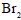in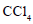forms
Test: Chemistry - 12 - Question 6
1-Methylcyclohexene is allowed to react with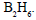The product is then treated with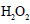and NaOH. The reaction is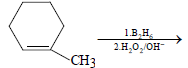The product formed is
Test: Chemistry - 12 - Question 7
By which of the following reactions can the conversion of cychlohexene (I) into 1,3-cyclohexadiene (III) be effected
Test: Chemistry - 12 - Question 8
Which of the following decolorizes the red-brown of a dilute solution ofin water ?
Test: Chemistry - 12 - Question 9
From which of the following hydrocarbons may hydrogen be displaced by a strongly electropositive
metal ?
Test: Chemistry - 12 - Question 10
When propyne is treated with aqueous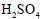,the major product formed is
Test: Chemistry - 12 - Question 11
Consider the following reaction sequence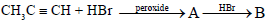The products (A) and (B) are, respectively
Test: Chemistry - 12 - Question 12
A hydrocarbon (A) is allowed to react with sodium amide and then with ethyl bromide to produce another hydrocarbon (B) which one ozonolysis produces propanoic acid only. The hydrocarbons (A) and (B) are, respectvely,
Test: Chemistry - 12 - Question 13
Acetylene does not react with
Test: Chemistry - 12 - Question 14
Which of the following hydrocarbons should be choosen as a starting material to prepare 3-hexanone by the hydration of the former ?
Test: Chemistry - 12 - Question 15
Chlorination of toluene in the presence of light and heat followed by treatement with aqueous NaOH and subsequently with dilute HCl gives
Test: Chemistry - 12 - Question 16
Among the compounds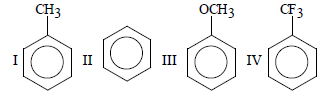the order of decreasing reactivity towards electrophilic substitution is
Test: Chemistry - 12 - Question 17
The function of anhydrous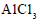in Fridel–Crafts reaction is to
Test: Chemistry - 12 - Question 18
The smallest alkane having a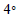carbon is
Test: Chemistry - 12 - Question 19
In which of the following reactions is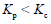?
Test: Chemistry - 12 - Question 20
For the equilibrium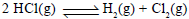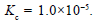If the equilibrium concentration of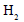and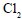is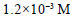and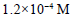respectively then the concentration of HCl is
Test: Chemistry - 12 - Question 21
In the reaction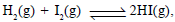the value of the equilibrium constant depends on
Test: Chemistry - 12 - Question 22
Consider the equilibrium,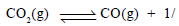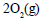The equilibrium constant K is given by (when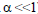)
Test: Chemistry - 12 - Question 23
1.7 g of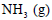is present in a 2-L flask. The active mass ofis
Test: Chemistry - 12 - Question 24
For the equilibrium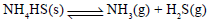Test: Chemistry - 12 - Question 25
For the eqilibrium,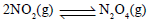+14.6 kcal increase in temperature would
Test: Chemistry - 12 - Question 26
When 1 mole of ethanol is mixed with 1 mole of acetic acid, 0.67 mole of ethyl acetate is produced at equilibrium. Calculate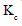for the reaction
Test: Chemistry - 12 - Question 27
During thermal dissoication, the observed vapour..........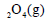is 26.0. Calculate the extent of
dissociation of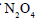(g)
Test: Chemistry - 12 - Question 28
When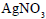is heated in a closed vessel,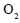gas is liberated and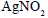is left behind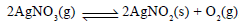,At equilibrium
Test: Chemistry - 12 - Question 29
For the heterogeneous equilibrium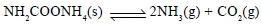the value of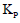is
Test: Chemistry - 12 - Question 30
The pH of a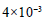M HCl solution is
Test: Chemistry - 12 - Question 31

The pH of a solution formed by maixing 40.0 mL of 0.1 M HCl with 10.0 mL of NaOH is

Detailed Solution for Test: Chemistry - 12 - Question 31

40ml  of 0.1MHCl
(0.1/1000)×40
= 0.004 moles of HCl
Similarly, 10ml of 0.45MNaOH
= (0.45)/1000 × 10
= 0.0045 moles of NaOH
Thus, NaOH left unneutralised = 0.0005 moles in a volume of 50ml
Concentration of NaOH = (1000×0.0005)/50=0.01
= 10-2M
Therefore, [OH-]=10-2M or [H+]
= 10-12M or pH = 12.0

Test: Chemistry - 12 - Question 32
The solubility of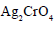is
Test: Chemistry - 12 - Question 33
The solubility product of CuS,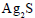and HgS are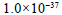,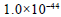and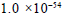respectively. The
solubilities of these suophides are in the order
Test: Chemistry - 12 - Question 34
When equal volumes of the following solutions are mixed, the precipitation of AgCl will occur only with (given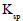of Agcl=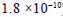)
Test: Chemistry - 12 - Question 35
For a weak monoprotic acid, the pH of a given concentration is calculated by the equation
Test: Chemistry - 12 - Question 36
For a salt of a weak acid and a weak base
Test: Chemistry - 12 - Question 37
The solubility product of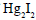is
Test: Chemistry - 12 - Question 38
Which of the following is arranged in order of increasing density ?
Test: Chemistry - 12 - Question 39
Which of the following alkali-metal hydroxides is the least soluble in water ?
Test: Chemistry - 12 - Question 40
Glauber’s salt is represented by the formula
Test: Chemistry - 12 - Question 41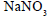being heated to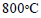gives
Test: Chemistry - 12 - Question 42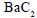reacts with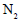to produce
Test: Chemistry - 12 - Question 43
Milk of lime is
Test: Chemistry - 12 - Question 44
Which of the following is arranged in order of decreasing solubility in water ?
Test: Chemistry - 12 - Question 45
Which of the following compounds is a perioxide

## NEET Mock Test Series

1 videos|25 docs|144 tests
 Use Code STAYHOME200 and get INR 200 additional OFF Use Coupon Code
Information about Test: Chemistry - 12 Page
In this test you can find the Exam questions for Test: Chemistry - 12 solved & explained in the simplest way possible. Besides giving Questions and answers for Test: Chemistry - 12, EduRev gives you an ample number of Online tests for practice

## NEET Mock Test Series

1 videos|25 docs|144 tests

### How to Prepare for NEET

Read our guide to prepare for NEET which is created by Toppers & the best Teachers